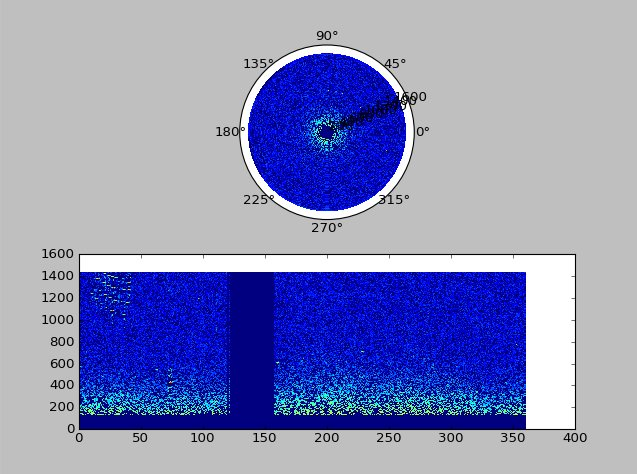# Polar plot of radar data

Hi all,

I’m trying to plot some radar data I’m working on, which is presented as a 2048x1440 2D array of ints. This means 2048 azimuth angle values between 0° and 360°, and 1440 range increments. Array values represent signal return in that angle/range coordinate. Due to hardware problems, some angle ranges are missing, there the signal value is zero in the grid.

The obvious way of plotting this would be a polar grid, so I used a pcolormesh, like this:
*import numpy as np
import matplotlib.pyplot as plt···

plt.ion()

fig = plt.figure()
theta,rad = np.meshgrid(np.arange(0., 360., 360./scan.shape), np.arange(scan.shape))
plt.draw()

I transposed scan because theta and rad are 1440x2048 arrays, where scan is a 2048x1440 array.

The resulting image seemed ok, but there were no gaps, which I knew were there, so I ran the same code without the ‘polar’ option, and put them side to side. The result is this:

​In the rectangular plot, there is a gap between around 120° to 160°, which should be an empty wedge in the polar plot, but it isn’t there. Same for the obvious echos at long range between 0° and 50°. OTOH the plot seems quite right, with the gating gap around the radar station in the center and the stronger echos at short range.

So I’m a bit baffled as to why this doesn’t work the way it should. Does anyone here have an explanation?

Alex Borghgraef

It is because the polar projection expects theta to be in radians, not degrees. This is a somewhat common mistake as the default labelling of a polar plot is in degrees, and so it tends to confuse new users.

Also, don’t forget that your radar data is (most likely) describing “bearing” (so, 0 azimuth is North rather than East).

Cheers!

Ben Root···

On Wed, Jun 25, 2014 at 9:14 AM, Alexander Borghgraef <alexander.borghgraef.rma@…287…> wrote:

Hi all,

I’m trying to plot some radar data I’m working on, which is presented as a 2048x1440 2D array of ints. This means 2048 azimuth angle values between 0° and 360°, and 1440 range increments. Array values represent signal return in that angle/range coordinate. Due to hardware problems, some angle ranges are missing, there the signal value is zero in the grid.

The obvious way of plotting this would be a polar grid, so I used a pcolormesh, like this:
*import numpy as np
import matplotlib.pyplot as plt
*

plt.ion()

fig = plt.figure()
theta,rad = np.meshgrid(np.arange(0., 360., 360./scan.shape), np.arange(scan.shape))
plt.draw()

I transposed scan because theta and rad are 1440x2048 arrays, where scan is a 2048x1440 array.

The resulting image seemed ok, but there were no gaps, which I knew were there, so I ran the same code without the ‘polar’ option, and put them side to side. The result is this:

​In the rectangular plot, there is a gap between around 120° to 160°, which should be an empty wedge in the polar plot, but it isn’t there. Same for the obvious echos at long range between 0° and 50°. OTOH the plot seems quite right, with the gating gap around the radar station in the center and the stronger echos at short range.

So I’m a bit baffled as to why this doesn’t work the way it should. Does anyone here have an explanation?

Alex Borghgraef

Open source business process management suite built on Java and Eclipse

Turn processes into business applications with Bonita BPM Community Edition

Quickly connect people, data, and systems into organized workflows

Winner of BOSSIE, CODIE, OW2 and Gartner awards

http://p.sf.net/sfu/Bonitasoft

Matplotlib-users mailing list

Matplotlib-users@lists.sourceforge.net

https://lists.sourceforge.net/lists/listinfo/matplotlib-users